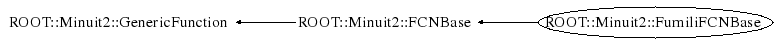# class ROOT::Minuit2::FumiliFCNBase: public ROOT::Minuit2::FCNBase

```

Extension of the FCNBase for the Fumili method. Fumili applies only to
minimization problems used for fitting. The method is based on a
linearization of the model function negleting second derivatives.
User needs to provide the model function. The figure-of-merit describing
the difference between the model function and the actual measurements
has to be implemented by the user in a subclass of FumiliFCNBase.
For an example see the FumiliChi2FCN and FumiliStandardChi2FCN classes.

@author  Andras Zsenei and Lorenzo Moneta, Creation date: 23 Aug 2004

@see <A HREF="http://www.cern.ch/winkler/minuit/tutorial/mntutorial.pdf">MINUIT Tutorial</A> on function minimization, section 5

@see FumiliChi2FCN

@see FumiliStandardChi2FCN

@ingroup Minuit

```

## Function Members (Methods)

This is an abstract class, constructors will not be documented.
Look at the header to check for available constructors.

public:
 virtual ~FumiliFCNBase() virtual unsigned int Dimension() virtual double ROOT::Minuit2::FCNBase::ErrorDef() const virtual void EvaluateAll(const vector& par) virtual const vector& Gradient() const virtual double Hessian(unsigned int row, unsigned int col) const virtual double ROOT::Minuit2::FCNBase::operator()(const vector& x) const ROOT::Minuit2::FCNBase& ROOT::Minuit2::FCNBase::operator=(const ROOT::Minuit2::FCNBase&) virtual void ROOT::Minuit2::FCNBase::SetErrorDef(double) virtual double ROOT::Minuit2::FCNBase::Up() const virtual double Value() const
protected:
 vector& Gradient() vector& Hessian() virtual void InitAndReset(unsigned int npar) void SetFCNValue(double Value)

## Data Members

private:
 vector fGradient vector fHessian const ROOT::Minuit2::ParametricFunction* fModelFunction unsigned int fNumberOfParameters double fValue

## Class Charts## Function documentation

virtual ~FumiliFCNBase()
```   FumiliFCNBase(const ParametricFunction& modelFCN) { fModelFunction = &modelFCN; }
```
`{}`
void EvaluateAll(const vector<double>& par)
```
Evaluate function Value, Gradient and Hessian using Fumili approximation, for values of parameters p
The resul is cached inside and is return from the FumiliFCNBase::Value ,  FumiliFCNBase::Gradient and
FumiliFCNBase::Hessian methods

@param par vector of parameters

```
double Value()
```Return cached Value of objective function estimated previously using the  FumiliFCNBase::EvaluateAll method

```
`{ return fValue; }`
```Return cached Value of function Gradient estimated previously using the  FumiliFCNBase::EvaluateAll method

```
`{ return fGradient; }`
double Hessian(unsigned int row, unsigned int col) const
```Return Value of the i-th j-th element of the Hessian matrix estimated previously using the  FumiliFCNBase::EvaluateAll method
@param row row Index of the matrix
@param col col Index of the matrix

```
unsigned int Dimension()
```return number of function variable (parameters) , i.e. function dimension

```
`{ return fNumberOfParameters; }`
void InitAndReset(unsigned int npar)
```initialize and reset values of gradien and Hessian

```
void SetFCNValue(double Value)
``` methods to be used by the derived classes to set the values
```
`{ fValue = Value; }`
`{ return fGradient; }`
`{ return fHessian; }`